﻿

### Zero-dimensional subschemes of projective spaces related to double points of linear subspaces and to fattening directions

#### Abstract

Fix a linear subspace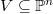$V\subseteq \mathbb {P}^n$ and a linearly independent set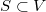$S\subset V$. Let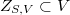$Z_{S,V} \subset V$ or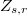$Z_{s,r}$ with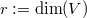$r:= \dim (V)$ and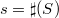$s=\sharp (S)$, be the zero-dimensional subscheme of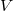$V$ union of all double points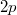$2p$,$p\in S$, of$V$ (not of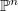$\mathbb {P}^n$ if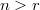$n>r$). We study the Hilbert function of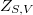$Z_{S,V}$ and of general unions in$\mathbb {P}^n$ of these schemes. In characteristic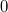$0$ we determine the Hilbert function of general unions of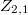$Z_{2,1}$ (easy), of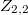$Z_{2,2}$ and, if$n=3$, general unions of schemes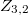$Z_{3,2}$ and$Z_{2,2}$

Keywords: zero-dimensional scheme; Hilbert function; postulation

Full Text: PDF